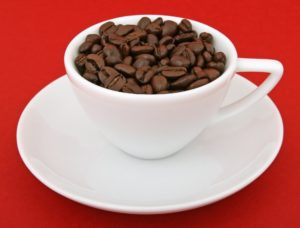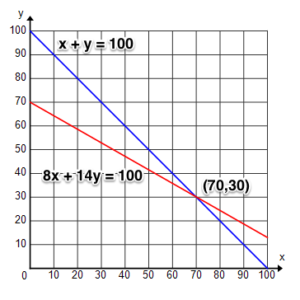## Putting It Together: Systems of Equations and Inequalities

You’ve come a long way since the beginning of the module when you were considering open your first coffee shop. Now that you know about systems of equations, you can solve your problem of how much of each type of coffee bean to put in your signature mixture.Recall that you were trying to make a 100-pound mixture of two kinds of coffee beans that would sell for $9.80 per pound. One bean sells for$8 per pound and the other sells for $14 per pound. You came up with two equations to describe your problem. Now you know that you have a system of equations. $\begin{gathered}x+y=100 \\ 8x+14y=980 \end{gathered}$ You have learned several different methods of solving a system of equations. Although all of the methods will work, you can help decide which one to use by looking at the equations. The first equation can easily be rearranged to solve for $x$ or $y$. You may find it easiest to do this, and then solve by substitution. Let’s choose $x$. Rearrange to solve for $x$. $x=100-y$ Now substitute for $x$ in the second equation, and solve for $y$.  Start with the original equation. $8x+14y=980$ Replace $x$ with $100-y$. $8\left(100-y\right)+14y=980$ Distribute. $800-8y+14y=980$ Combine like terms. $800+6y=980$ Subtract 800 from both sides. $6y=180$ Divide both sides by 6. $y=30$ Now that you know the value of $y$, you can solve for $x$.  Start with the original equation. $x+y=100$ Replace y with 30. $x+30=100$ Subtract 30 from both sides. $x=70$ The solution to the system of equations is (70, 30). What does this solution mean? Remember that you were looking for the number of pounds of each type of coffee bean. The solution indicates that you should use 70 pounds of the$8-beans and 30 pounds of the \$14-beans. Does this make sense?  Take a moment to check.

70 pounds + 30 pounds = 100 pounds

$70(8)+30(14)=980$

Yes, your answer makes sense. Is this the only possible combination? Making a graph is always a nice way to classify a system of equations to determine if there is only one solution. The graph below represents these equations.As you can see, the lines intersect at a single point, which is exactly the solution you found. This system of equations is independent. The only way the solution will change is if you choose different coffee beans at different prices or change the price per pound of the mixture. That is something you just might do as your business grows and you want to increase your profits. But for now, you might want to concentrate on making a reasonably priced mixture that will bring in new customers.